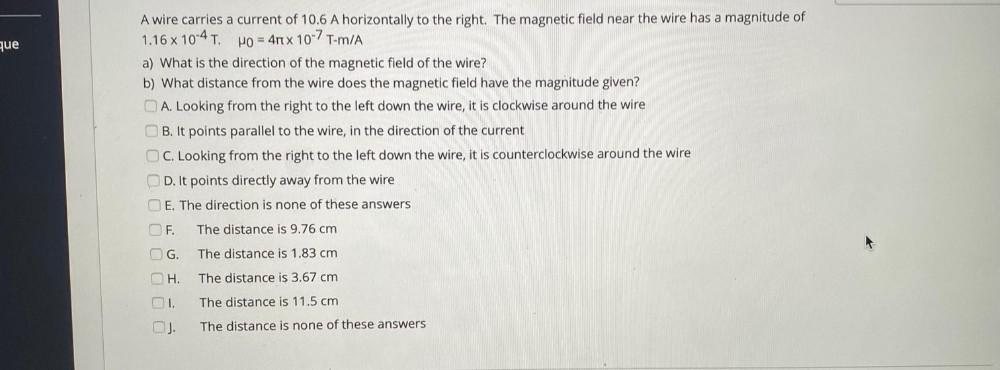Question:

# que A wire carries a current of 10.6 A horizontally to the right. The magnetic field near the wire has a magnitude of 1.16 x 10-que A wire carries a current of 10.6 A horizontally to the right. The magnetic field near the wire has a magnitude of 1.16 x 10-4 T. Ho = 4rt x 10-7 T-m/A a) What is the direction of the magnetic field of the wire? b) What distance from the wire does the magnetic field have the magnitude given? A. Looking from the right to the left down the wire, it is clockwise around the wire B. It points parallel to the wire, in the direction of the current OC. Looking from the right to the left down the wire, it is counterclockwise around the wire D. It points directly away from the wire E. The direction is none of these answers OF. The distance is 9.76 cm G. The distance is 1.83 cm H. The distance is 3.67 cm OI. The distance is 11.5 cm The distance is none of these answers